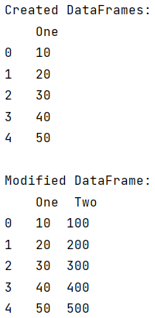# Make Pandas DataFrame apply() use all cores

Learn how to make Pandas DataFrame apply() use all cores?
Submitted by Pranit Sharma, on June 18, 2022

A core is a processor of a processor inside a computer system that is used for running the computer processes. Whenever we perform some operations, it is the core that performs the operation.

Nowadays, computers have multi-cores so that the process of computations becomes faster.

The pandas.DataFrame.apply() method is limited to single-core which means that these modern machines will only compute a single process at a time if apply() method is used.

To make pandas DataFrame apply() to all the cores, we will install a swifter package that efficiently applies any function to pandas DataFrame or Series in the fastest available manner.

To work with pandas, we need to import pandas package first, below is the syntax:

```import pandas as pd
```

Let us understand with the help of an example,

## Python code to make Pandas DataFrame apply() use all cores

```# Importing pandas package
import pandas as pd

# Importing swifter package
import swifter as sw

# Defining a function
def function(data):
return data * 10

# Creating a DataFrame
df = pd.DataFrame([10,20,30,40,50],columns=['One'])

# Display DataFrame with NaN values
print("Created DataFrames:\n",df,"\n")

# Applying swifter
df['Two'] = df['One'].swifter.apply(function)

# Display modified DataFrame
print("Modified DataFrame:\n",df)
```

Output:What's New (MCQs)

Top Interview Coding Problems/Challenges!

IncludeHelp's Blogs

Languages: » C » C++ » C++ STL » Java » Data Structure » C#.Net » Android » Kotlin » SQL
Web Technologies: » PHP » Python » JavaScript » CSS » Ajax » Node.js » Web programming/HTML
Solved programs: » C » C++ » DS » Java » C#
Aptitude que. & ans.: » C » C++ » Java » DBMS
Interview que. & ans.: » C » Embedded C » Java » SEO » HR
CS Subjects: » CS Basics » O.S. » Networks » DBMS » Embedded Systems » Cloud Computing
» Machine learning » CS Organizations » Linux » DOS
More: » Articles » Puzzles » News/Updates